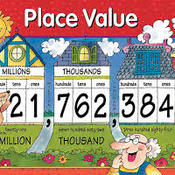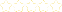• > Rachel Casto
• > Place Value, Addition, Subtraction
+Owner: Rachel Casto
##### Description:
Number and Operations in Base Ten 4.NBT  A. Generalize place value understanding for multi-digit whole numbers. 1. Recognize that in a multi-digit whole number, a digit in one place represents ten times what it represents in the place to its right. For example, recognize that 700 ÷ 70 = 10 by applying concepts of place value and division. 2. Read and write multi-digit whole numbers using base-ten numerals, number names, and expanded form. Compare two multi-digit numbers based on meanings of the digits in each place, using >, =, and < symbols to record the results of comparisons. 3.Use place value understanding to round multi-digit whole numbers to any place. B. Use place value understanding and properties of operations to perform multi-digit arithmetic. 4. Fluently add and subtract multi-digit whole numbers using the standard algorithm. (Cover art from lisdelemmath.blogspot.com)
6 Tutorials in this Playlist

#### Ways to Read and Write a Number

Author: Rachel Casto
Rating:(9)

#### Place Value

Author: Rachel Casto
Rating:(1)

#### Rounding Numbers

Author: Rachel Casto
Rating:(2)

#### Comparing Numbers

Author: Rachel Casto
Rating:(0)